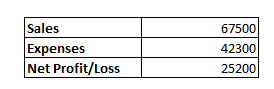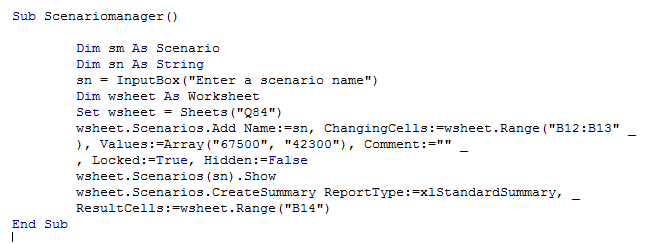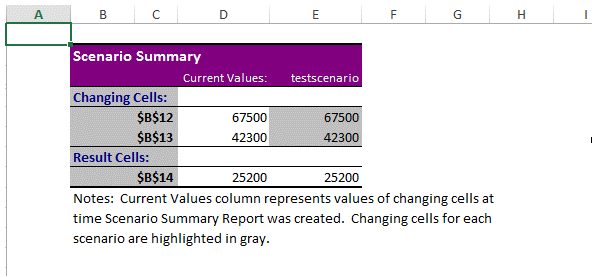## Vba scenario manager in vba in Excel

Scenario Manager comes under "What If" Analysis of Excel.

As the name indicates, it is a prediction tool that allows users to check output for various expected input values.

Scenario Manager is one among the "What If" Analysis tool that predicts the possible outcome of various scenarios.

A�Scenario�is a set of values that Excel saves and can substitute automatically on worksheet.

If there are several scenarios for which the outcome has to be predicted in order to choose a right plan, then the "Scenario Manager" can be of very much use.

Collect all possible values and substitute the values to see the possible output.

The following example illustrates this:

Financial Prediction 2017The following code shows how to create a Scenario Manager in VBA.

Sub Scenariomanager()

Dim sm As Scenario

Dim sn As String

sn = InputBox("Enter a scenario name")

Dim wsheet As Worksheet

Set wsheet = Sheets("Q84")

), Values:=Array("67500", "42300"), Comment:="" _

, Locked:=True, Hidden:=False

wsheet.Scenarios(sn).Show

wsheet.Scenarios(sn).Show

wsheet.Scenarios(sn).Show

wsheet.Scenarios(sn).Show

Note that, the scenario name has to be different, every time the code is tested,else, it will result in an error.

The screenshot of the editor and the output is as shown below.